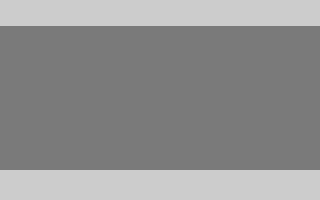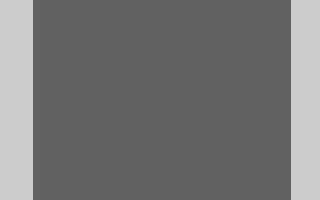# Usage¶

Using thumbor is really straightforward. thumbor offers one endpoint for retrieving the image and a very similar endpoint to retrieve metadata.

## Image Endpoint¶

/hmac/trim/AxB:CxD/(adaptive-)(full-)fit-in/-Ex-F/HALIGN/VALIGN/smart/filters:FILTERNAME(ARGUMENT):FILTERNAME(ARGUMENT)/*IMAGE-URI*

• hmac is the signature that ensures Security ;
• trim removes surrounding space in images using top-left pixel color unless specified otherwise;
• AxB:CxD means manually crop the image at left-top point AxB and right-bottom point CxD;
• fit-in means that the generated image should not be auto-cropped and otherwise just fit in an imaginary box specified by ExF. If a full fit-in is specified, then the largest size is used for cropping (width instead of height, or the other way around). If adaptive fit-in is specified, it inverts requested width and height if it would get a better image definition;
• -Ex-F means resize the image to be ExF of width per height size. The minus signs mean flip horizontally and vertically;
• HALIGN is horizontal alignment of crop;
• VALIGN is vertical alignment of crop;
• smart means using smart detection of focal points;
• filters can be applied sequentially to the image before returning;
• IMAGE-URI is the encoded URI for the image you want resized.

### Trim¶

Removing surrounding space in images can be done using the trim option.

Unless specified trim assumes the top-left pixel color and no tolerance (more on tolerance below).

To use it, just add a /trim part to your URL.

If you need to specify the orientation from where to get the pixel color, just use /trim:top-left for the top-left pixel color or /trim:bottom-right for the bottom-right pixel color.

Trim also supports color tolerance. The euclidean distance between the colors of the reference pixel and the surrounding pixels is used. If the distance is within the tolerance they’ll get trimmed. For a RGB image the tolerance would be within the range 0-442.

### Manual Crop¶

The manual crop is entirely optional. This is very useful for applications that provide custom real-time cropping capabilities to their users.

The manual crop part of the url takes two points as arguments, separated by a colon. The first point is the left-top point of the cropping rectangle. The second point is the right-bottom point.

This crop is performed before the rest of the operations, so it can be used as a prepare step before resizing and smart-cropping. It is very useful when you just need to get that celebrity face on a big picture full of people, as an example.

### Fit in¶

The fit-in argument specifies that the image should not be auto-cropped and auto-resized to be EXACTLY the specified size, and should be fit in an imaginary box of “E” width and “F” height, instead.

Consider an image of $$800px$$ x $$600px$$, and a fit of $$300px$$ x $$200px$$. This is how thumbor would resize it:Consider an image of $$400px$$ x $$600px$$, and a fit of $$300px$$ x $$200px$$. This is how thumbor would resize it:This is very useful when you need to fit an image somewhere, but you have no idea about the original image dimensions.

If a full fit-in is used, instead of using the largest size for cropping it uses the smallest one, so in the above scenarios:

For the image of $$800px$$ x $$600px$$, with a full fit-in of $$300px$$ x $$200px$$, we would get an image of $$300px$$ x $$225px$$.

For the image of $$400px$$ x $$600px$$, with a full fit-in of $$300px$$ x $$200px$$, we would get an image of $$300px$$ x $$450px$$.

### Image Size¶

The image size argument specifies the size of the image that will be returned by the service. Thumbor uses smart Crop and Resize Algorithms

If you omit one of the dimensions or use zero as a value (as in $$300x$$, $$300x0$$, $$x200$$, $$0x200$$, and so on), Thumbor will determine that dimension as to be proportional to the original image. Say you have an $$800x600$$ image and ask for a $$400x0$$ image. Thumbor will infer that since $$400$$ is half of $$800$$, then the height you are looking for is half of $$600$$, which is $$300px$$.

If you use $$0x0$$, Thumbor will use the original size of the image and thus won’t do any cropping or resizing.

If you specify one of the dimensions as the string “orig” (as in $$origx100$$, $$100xorig$$, $$origxorig$$), thumbor will interpret that you want that dimension to remain the same as in the original image. Consider an image of $$800x600$$. If you ask for a $$300xorig$$ version of it, thumbor will interpret that you want a $$300x600$$ image. If you instead ask for a $$origx300$$ version, thumbor will serve you an $$800x300$$ image.

If you use $$origxorig$$, Thumbor will use the original size of the image and thus won’t do any cropping or resizing.

The default value (in case it is omitted) for this option is to use proportional size (0) to the original image.

### Horizontal Align¶

As was explained above, unless the image is of the same proportion as the desired size, some cropping will need to occur.

The horizontal align option controls where the cropping will occur if some width needs to be trimmed (unless some feature detection occurs - more on that later).

So, if we need to trim $$300px$$ of the width and the current horizontal align is “left”, then we’ll trim 0px of the left of the image and $$300px$$ of the right side of the image.

The possible values for this option are:

• left - only trims the right side;
• center - trims half of the width from the left side and half from the right side;
• right - only trims the left side.

It is important to notice that this option is useless in case of the image being vertically trimmed, since Thumbor’s cropping algorithm only crops in one direction.

The default value (in case it is omitted) for this option is “center”.

### Vertical Align¶

The vertical align option is analogous to the horizontal one, except that it controls height trimming.

So, if we need to trim $$300px$$ of the height and the current vertical align is “top”, then we’ll trim $$0px$$ of the top of the image and $$300px$$ of the bottom side of the image.

The possible values for this option are:

• top - only trims the bottom;
• middle - trims half of the height from the top and half from the bottom;
• bottom - only trims the top.

It is important to notice that this option is useless in case of the image being horizontally trimmed, since Thumbor’s cropping algorithm only crops in one direction.

The default value (in case it is omitted) for this option is “middle”.

### Smart Cropping¶

Thumbor uses some very advanced techniques for obtaining important points of the image (referred to as Focal Points in the rest of this documentation).

Even though Thumbor comes with facial recognition of Focal Points as well as feature recognition, you can easily implement your own detectors as you’ll see further in the docs.

There’s not much to this option, since we’ll cover it in the Detection Algorithms page. If you use it in the url, smart cropping will be performed and will override both horizontal and vertical alignments if it finds any Focal Points.

The default value (in case it is omitted) for this option is not to use smart cropping.

### Filters¶

Thumbor allows for usage of a filter pipeline that will be applied sequentially to the image. Filters are covered in the Filters page if you want to know more.

To use filters add a filters: part in your URL. Filters are like function calls filter_name(argument, argument2, etc) and are separated using the : character, like filters:filter_name():other_filter().

### Image URI¶

This is the image URI. The format of this option depends heavily on the image loader you are using. Thumbor comes pre-packaged with an HTTP loader and a Filesystem loader.

If you use the HTTP loader, this option corresponds to the image complete URI.

If you use the Filesystem loader, this option corresponds to the path of the image from the images root.

The metadata endpoint has ALL the options that the image one has, but instead of actually performing the operations in the image, it just simulates the operations.

Since it has the same options as the other endpoint, we won’t repeat all of them. To use the metadata endpoint, just add a /meta in the beginning of the url.

Say we have the following crop URL:

http://my-server.thumbor.org/unsafe/-300x-200/left/top/smart/path/to/my/nice/image.jpg

If we want the metadata on what thumbor would do, just change the url to be

http://my-server.thumbor.org/unsafe/meta/-300x-200/left/top/smart/path/to/my/nice/image.jpg

After the processing is finished, thumbor will return a json object containing metadata on the image and the operations that would have been performed.

The json looks like this:

{
thumbor: {
source: {
url: "path/to/my/nice/image.jpg",
width: 800,
height: 600
},
operations: [
{
type: "crop",
left: 10,
top: 10,
right: 300,
bottom: 200
},
{
type: "resize",
width: 300,
height: 200
},
{ type: "flip_horizontally" },
{ type: "flip_vertically" }
]
}
}